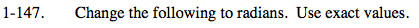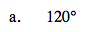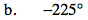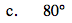### Home > PC > Chapter 1 > Lesson 1.4.2 > Problem1-147

1-147.Make a ratio comparing a half circle in degrees and radians.

$\frac{\pi\ \text{radians}}{180\degree} = \frac{x}{120\degree}$

$120\degree\ \frac{\pi\ \text{radians}}{180\degree} =x$

$\frac{2}{3}\ \pi\text{ radians} = x$Follow a similar process as (a).Follow a similar process as (a).